## The Discrete Fourier Transform

Let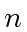be a positive integer and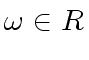be a primitive-th root of unity. In what follows we identify every univariate polynomial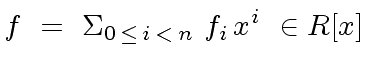(13)

of degree less thanwith its coefficient vector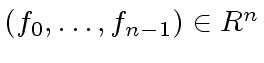.

Definition 2   The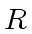-linear map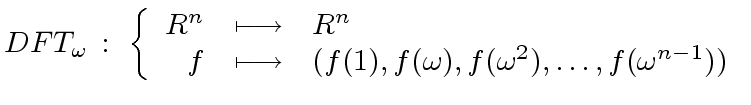(14)

which evaluates a polynomial at the powers of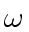is called the Discrete Fourier Transform (DFT).

Proposition 2   The-linear map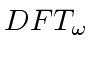is an isomorphism.

Proof. Since the-linear mapis an endomorphism (the source and target spaces are the same) we only need to prove thatis bijective. Observe that the Vandermonde matrix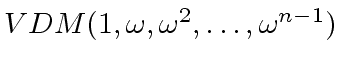is the matrix of the-linear map. Then for proving thatis bijective we need only to prove thatis invertible which holds iff the values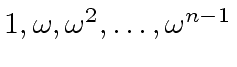are pairwise different. A relation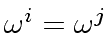for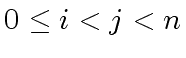would imply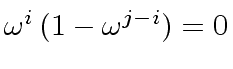. Since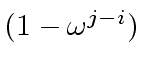cannot be zero or a zero divisor then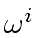and thusmust be zero. Thencannot be a root of unity. A contradiction. Therefore the valuesare pairwise different andis an isomorphism.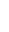Proposition 3   Letdenote the matrix of the isomorphism. Then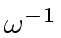the inverse ofis also a primitive-th root of unity and we have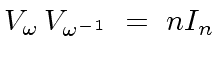(15)

where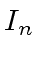denotes the unit matrix of order.

Proof. Define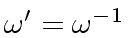. Observe that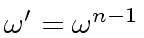. Thus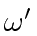is a root of unity. We leave to the reader the proof that this a primitive-th root of unity,

Let us consider the product of the matrixand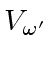. The element at row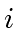and column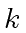is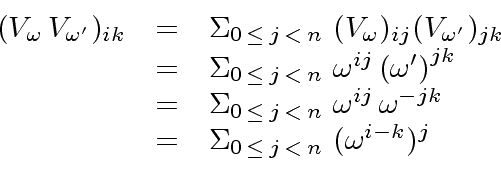(16)

Observe that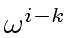is either a power ofor a power of its inverse. Thus, in any case this is a power of. If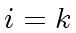this power is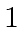and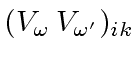is equal to. If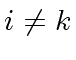then the conclusion follows by applying the second statement of Lemma 1 which shows that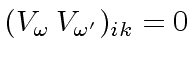.Marc Moreno Maza
2008-01-07